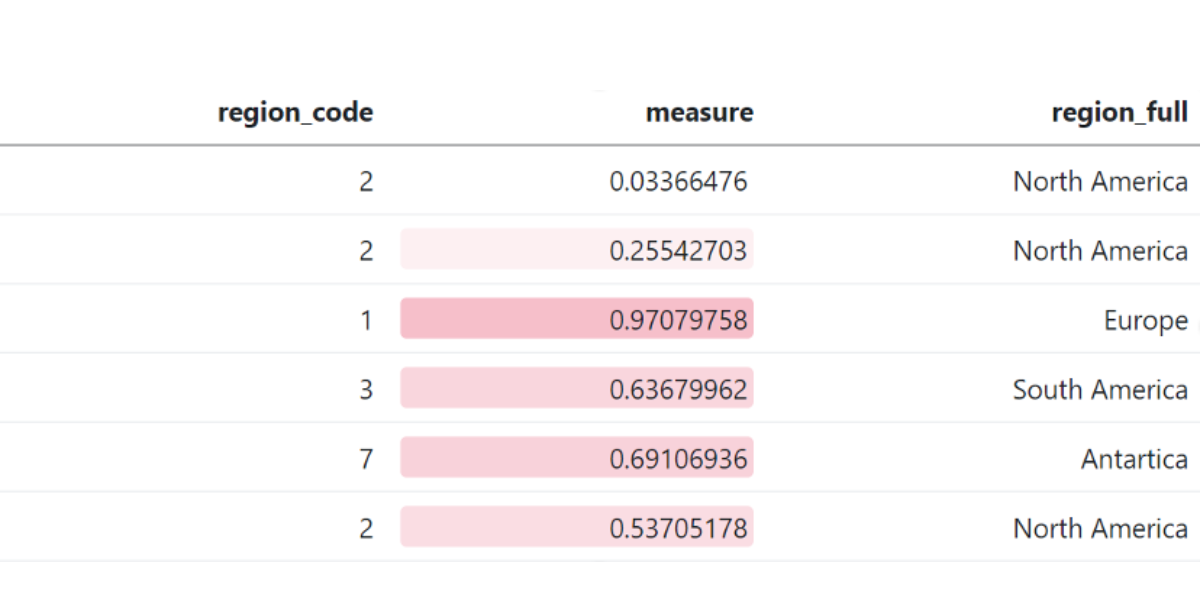# Mapping SAS Formats to R, Part 2 – A Comprehensive Guide for the SAS Programmer

• SAS
• R
##### 1. Picking Up From Last Time

Last time, we introduced the SAS format and looked at methods for replicating two aspects of it’s functionality in R, specifically performing a lookup and grouping data. Today, we’ll investigate how to:

• Apply a “mask” to data. This means that you can change the way that data is displayed, without changing the underlying data (e.g. a value of -0.6534 could print as (65.3%))
• Read formatted data as a different type, for example to read a value of “£1,000.00” in a CSV as 1000.

Using a format as a “mask” for data is the most common use-case in SAS, keeping the underlying data the same but applying formatting when it is displayed. There are a number of methods we can use to replicate this in R.

###### 2.1 Method 1: Base R

Using Base R, there are several different functions that can format data.

The first we’ll use is the format() function, which can format any R object for printing, but is typically used for numeric vectors and has a large set of options:

```numbers <- runif(10, 1-1000000, 1000000)

format(numbers, justify="right", width="10", big.mark=",") |> print()

#>  "-424,844.96" " 576,610.27" "-182,046.16" " 766,034.81" " 880,934.57"
#>  "-908,887.00" " 56,210.98" " 784,838.09" " 102,870.03" " -86,770.53"

format(numbers, justify=3, scientific=TRUE) |> print()
#>  "-4.25e+05" " 5.77e+05" "-1.82e+05" " 7.66e+05" " 8.81e+05" "-9.09e+05"
#>  " 5.62e+04" " 7.85e+05" " 1.03e+05" "-8.68e+04"

```

Alternatively, the sprintf function provides a familiar interface to those used to C style formatting, for more detail run ?sprintf.

```sprintf("\$%.2f", numbers)

#>   "\$-424844.96" "\$576610.27"  "\$-182046.16" "\$766034.81"  "\$880934.57"
#>   "\$-908887.00" "\$56210.98"   "\$784838.09"  "\$102870.03"  "\$-86770.53"

```
###### 2.2 Method 2: {scales}

The {scales} package is used by {ggplot2} to scale data for graphs, but it also includes some useful formatting functions that can help with common formats. For example:

```scales::label_dollar(prefix="£", style_negative="parens")(numbers)

#>  "(£424,845)" "£576,610" "(£182,046)" "£766,035" "£880,935"

#>  "(£908,887)" "£56,211" "£784,838" "£102,870" "(£86,771)"
```

Note that all the scale::label_… functions return another function, and we’ve passed the numbers vector into this. There are label functions for currency, percents, dates, time, mathematics, SI units, p-values, and more! If a particular label is used frequently in your code, it may be helpful to assign it to a helper function

```percent <- scales::label_percent(
accuracy=0.1,
suffix="%",
decimal.mark=",",
style_negative="parens"
)

percent(runif(5, -1, 1))

#>  "91,4 %" "(9,3 %)" "35,5 %" "14,5 %" "(79,4 %)"```
###### 2.3 Method 3: {formattable}

All the examples covered so far have one key difference to SAS formats, they return the data converted to a new format, rather than the original data with a mask that applies when printing. For this, we can use {formattable}. This lets us apply formats to a vector or data.frame, and then continue to work with them as if they were standard R vectors. Only when it comes time to print does the format get applied.

For example:

```values <- (runif(5, -1, 1)

percs <- formattable::percent(values)

print(percs)#>  79.96% -50.78% -91.59% -34.42% 90.90%

print(percs**2)

#>  63.94% 25.79% 83.88% 11.84% 82.63%```

This is not limited to standard numeric formats, for which {formattable} includes methods for: digits, commas, percents, accounting, currency, and scientific notation. There are also advanced methods for styling the output table, for example conditional formatting similar to Excel:

```
my_data <- data.frame(
region_code = c("SA", "SA", "E", "AS", "AN", "NA"),
measure = runif(6)
)
formattable::formattable(
my_data,
list(measure=formattable::color_tile("white", "pink"))
)```Note however that {formattable} formats will not be persisted unless the data is saved in an Rdata or RDS format.

The final use of a SAS (in)format that we’d like to replicate is reading in character data as numeric. The simplest way to do this is using parse_number from {readr}, which provides a method to ignore non-numeric data in a column and attempt to parse it as a number:

```readr::parse_number(c("£1,000.00"))
#>  1000```

And for date formats, {readr} also provides readr::parse_date or readr::parse_datetime. Each of these functions should generally not be used explicitly, instead use the col_types argument of readr::read_csv and similar to specify that a column is datetime, date, numeric, etc.

However, when it comes to more complex formats (e.g. “(82.5%)”) this will not take into account that it is a percentage and negative, and so more custom methods must be used:

```values <- c("(82.5%)", "2%", "28.55%")

my_format <- function(x){
x |>
stringr::str_replace_all(c("\\("" = "-", "\\)" = "")) |>